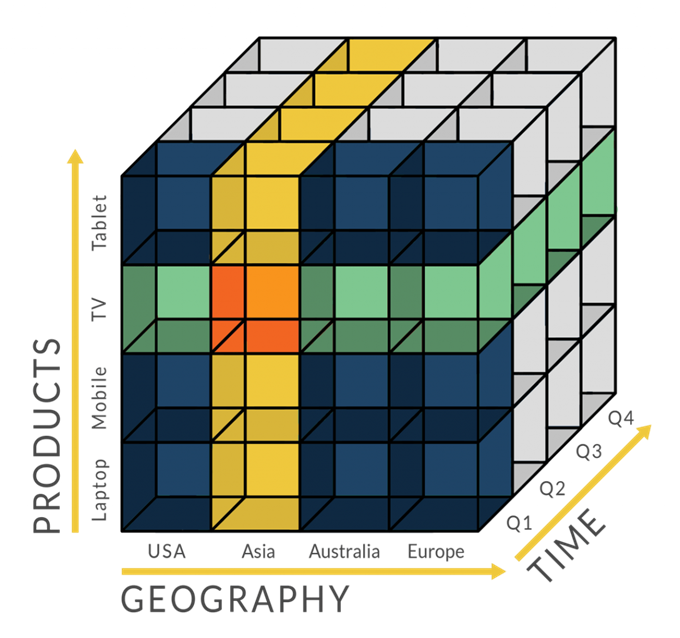# 贝塞尔曲线与B样条曲线

## 贝塞尔曲线分类

• 一阶贝塞尔曲线（2 个控制点）
• 二阶贝塞尔曲线（3 个控制点）
• 三阶贝塞尔曲线（4 个控制点）
• n阶贝塞尔曲线（n+1个控制点）

## 如何绘制贝塞尔曲线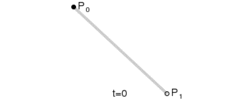$$B_1(t)=P_0 + (P_1 – P_0)t$$

$$B_1(t)=(1-t)P_0 + tP_1,t\in [0,1]$$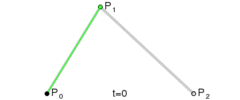$$P_0^{‘} = (1 – t)P_0 + tP_1$$

$$P_1^{‘} = (1 – t)P_1 + tP_2$$

$$B_{2}(t) = (1 – t)P_0^{‘} + tP_1^{‘}$$

$$= (1 – t)((1 – t)P_0 + tP_1) + t((1 – t)P_1 + tP_2)$$

$$= (1 – t)^2P_0 + 2t(1 – t)P_1 + t^2P_2$$

$$B_{2}(t) = (1 – t)^2P_0 + 2t(1 – t)P_1 + t^2P_2 , t\in[0, 1]$$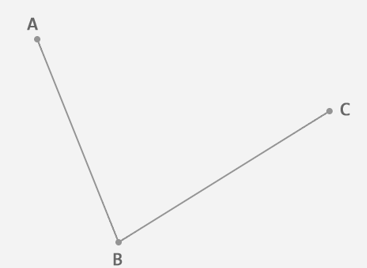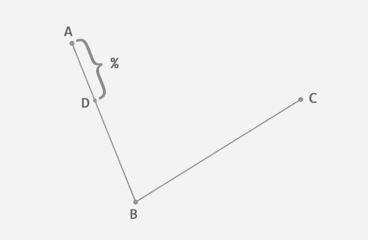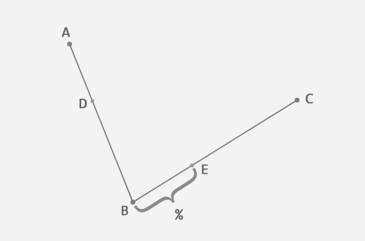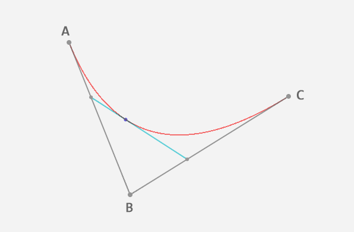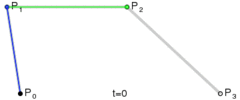$$B_{3}(t) = (1 – t)^3P_0 + 3t(1 – t)^2P_1 + 3t^2(1 – t)P_2 + t^3P_3 , t\in[0, 1]$$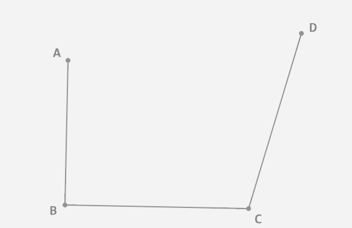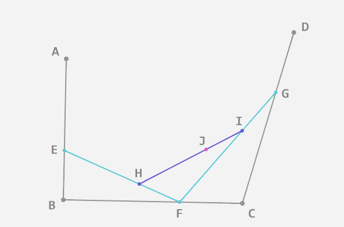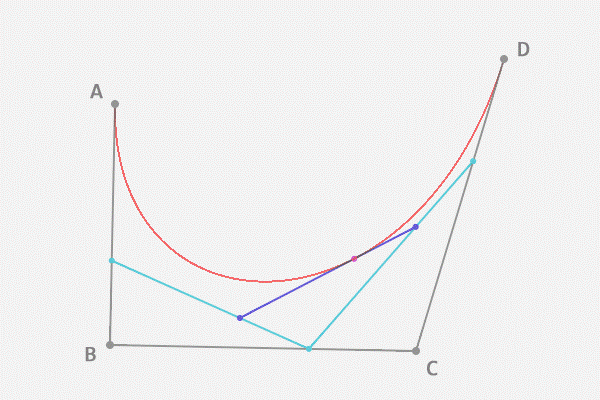n阶贝塞尔曲线公式：

$$B(t) = \sum_{i=0}^{n}C_n^{i}P_i(1-t)^{n-i}t^i,t\in[0,1]$$

$$B(t) = \sum_{i=0}^{n}P_ib_{i,n}(t),t\in[0,1]$$

$$b_{i,n}(t)=C_n^{i}(1-t)^{n-i}t^i,其中i=0,1,…,n$$

## 什么是样条？

• 从力学角度考虑，样条可看作一弹性细梁，压铁是作用在梁上的集中载荷。由此，设计样条曲线的过程可抽象为：求弹性细梁在外加集中载荷作用下产生的弯曲变形。
• 在数学上使用分段的三次多项式函数来描绘这种曲线，其中各曲线段的连接处有连续的一次和二次导数。
• 在计算机图形学中，样条曲线指由多项式曲线段连接而成的曲线，在每段的边界处满足特定的连续性条件。

## 什么是B样条？

• 确定了多边形的顶点数（m个），也就决定了所定义的Bezier曲线的阶次（m-1次），这样很不灵活。
• 当顶点数（m）较大时，曲线的阶次将比较高。此时，多边形对曲线形状的控制将明显减弱。
• Bezier的调和函数的值，在开区间（0,1）内均不为0。因此，所定义的曲线在（0<t<1）的区间内的任何一点均要受到全部顶点的影响，即改变其中任一个顶点的位置，都将对整条曲线产生影响，因此对曲线进行局部修改是不可能的。

B样条曲线，简单来说，它是对贝塞尔曲线的一个补充。为什么这样说呢？是因为贝塞尔曲线某些情况下不实用：曲线上每个点受所有控制点影响，这会给调整曲线工作带来麻烦。可以想到的第一个优化是，把整个贝塞尔曲线变成多段贝塞尔子曲线的拼接。然而，这个方案也不好用，因为拼接工作很难做好，因为要拼接曲线显得“光滑”前提是保证相邻曲线之间的连续性。

B样条是对贝塞尔曲线的一般化。因而，Bezier曲线能表达的，B样条也同样可以。而且，因为B样条引入了控制点个数与曲线阶次无关的特性，使得我们可以对低阶曲线有了更好的控制能力。而且，通过对节点序列的控制，使得我们对曲线有自由的调节能力。因此，B样条曲线在外形设计中得到了更广泛的重视和应用。

• B样条的控制点个数与曲线次数无关，因此B样条的自由度更大，可以定义很多控制点又不用担心曲线次数过高而计算困难。
• B样条有多个节点区间，使得其为分段函数，而Bezier曲线只有一个区间。

B样条取消与赛贝尔曲线的关系

• B样条上任意一个区间$[u_i,u_{i+1})$上的曲线的定义都是一个Bezier。因此可以将B样条转为分段bezier曲线。
• Bezier是一种特殊的样条曲线。

## 样条及B样条再Python中的使用

import numpy as np
import matplotlib.pyplot as plt
from scipy.interpolate import spline

x = range(50)
y = [137, 17, 110, 383, 477, 623, 858, 1165, 1353, 1516, 2039, 1353, 2453, 1245, 2136, 1149, 1148, 894, 277, 414, 399, 297, 103, 102, 103, 57, 54, 63, 52, 27,38, 102, 14, 13, 31, 38, 11, 17, 51, 11, 19, 14, 22, 18, 29, 3, 7, 6, 11, 142]

x = np.array(x)
y= np.array(y)

x_smooth = np.linspace(x.min(),x.max(),1000)
y_smooth = spline(x, y, x_smooth)

plt.plot(x_smooth, y_smooth)
plt.show()


B样条示例代码：

import numpy as np
import matplotlib.pyplot as plt
from scipy.interpolate import BSpline, splrep

x = range(50)
y = [137, 17, 110, 383, 477, 623, 858, 1165, 1353, 1516, 2039, 1353, 2453, 1245, 2136, 1149, 1148, 894, 277, 414, 399,
297, 103, 102, 103, 57, 54, 63, 52, 27, 38, 102, 14, 13, 31, 38, 11, 17, 51, 11, 19, 14, 22, 18, 29, 3, 7, 6, 11,
142]

x = np.array(x)
y = np.array(y)

t, c, k = splrep(x, y, s=0, k=3)

x_smooth = np.linspace(x.min(), x.max(), 1000)
y_smooth = BSpline(t, c, k, extrapolate=False)

plt.plot(x, y, 'ko', label='Original points')
plt.plot(x_smooth, y_smooth(x_smooth), 'r', label='BSpline')
plt.legend(loc='best')
plt.show()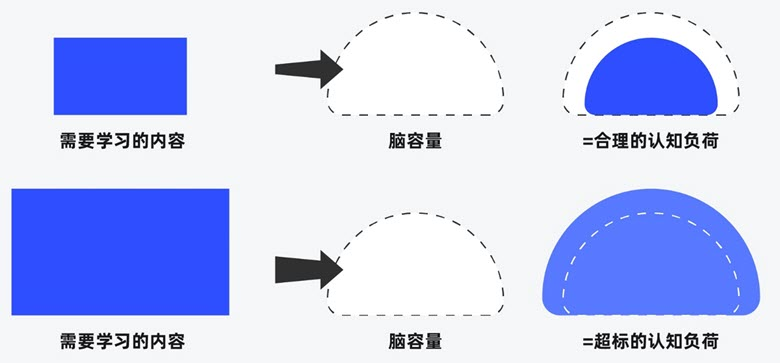##### 界面设计和游戏设计中的认知负荷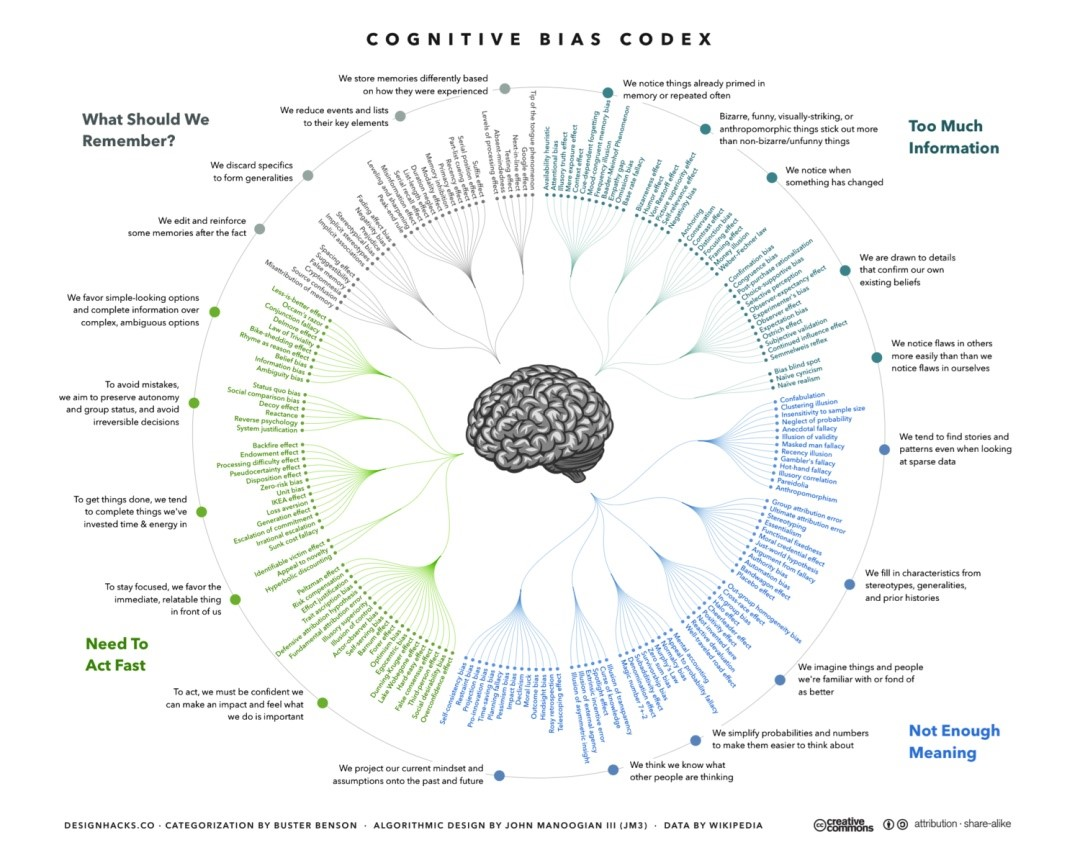##### 理解认知偏差